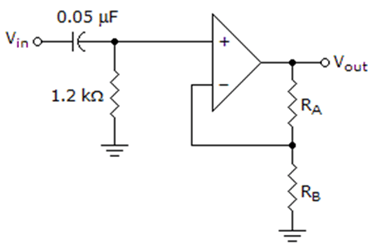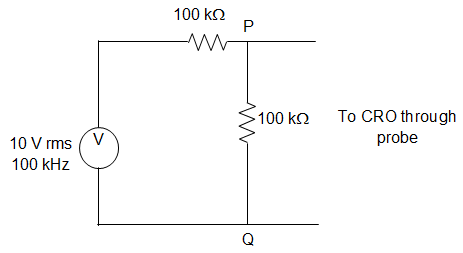Q.1
##### A voltage having the waveform of a sine curve is applied across a capacitor. When the frequency of the voltage is increased, what happens to the current through the capacitor?
• a) Increases
• b) Decreases
• c) Remains same
• d) Is zero
Q.2
• a) 40 Hz
• b) 200 Hz
• c) 250 Hz
• d) 1250 Hz
Q.3
• a) 3.53 V
• b) 3.47 V
• c) 5.54 V
• d) 7.00 V
Q.4
##### A signal e-at sin (ωt) is the input to a real linear time invariant system. Given K and ∅ are constants, the output of the system will be of the form Ke-bt sin (vt + ∅). The correct statement among the following is __________
• a) b need not be equal to a but v must be equal to ω
• b) v need not be equal to ω but b must be equal to a
• c) b must be equal to a and v must be equal to ω
• d) b need not be equal to a and v need not be equal to ω
Q.5
##### Let us suppose that the impulse response of a causal LTI system is given as h (t). Now, consider the following two statements:• a) Statement 1 is correct and Statement 2 is wrong
• b) Statement 2 is correct and Statement 1 is wrong
• c) Both Statement 1 and Statement 2 are wrong
• d) Both statement 1 and Statement 2 are correct
Q.6
##### For which of the following a Fourier series cannot be defined?• a) 3 sin (25t)
• b) 4 cos (20t + 3) + 2 sin (710t)
• c) exp(-|t|) sin (25t)
• d) 1
Q.7
• a) 1
• b) 0.5
• c) 2
• d) 2.5